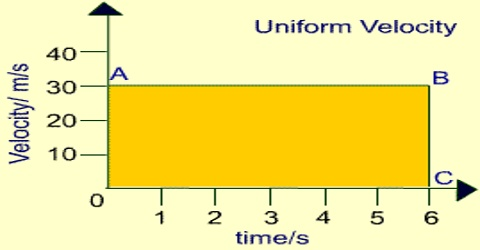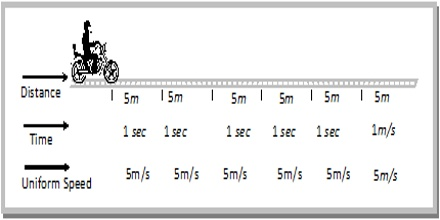# Uniform Velocity Related to Motion

Uniform velocity

If the velocity always remains constant then the velocity is called uniform velocity. an object with uniform velocity continues to cover the same amount of distance over the same time interval without changing its direction.

If magnitude and direction of the force applied on a body are constant, then velocity also remains constant. This velocity of the body is the uniform velocity.

Example: Velocity of sound, velocity of light etc.

Explanation: In figure (a) position of a moving body along a straight line has been shown by five successive points with 1 second interval. Here the distance between two successive points is 0.5 m. According to motion the body is traveling 0.5 m in every second in the same direction and traveling same path in the same interval of time.figure (a)

So, the velocity of the body is uniform velocity and the magnitude of that uniform velocity is 0.5 ms-1. In figure (b), uniform velocity has been shown by a graph of displacement versus time.figure (b)